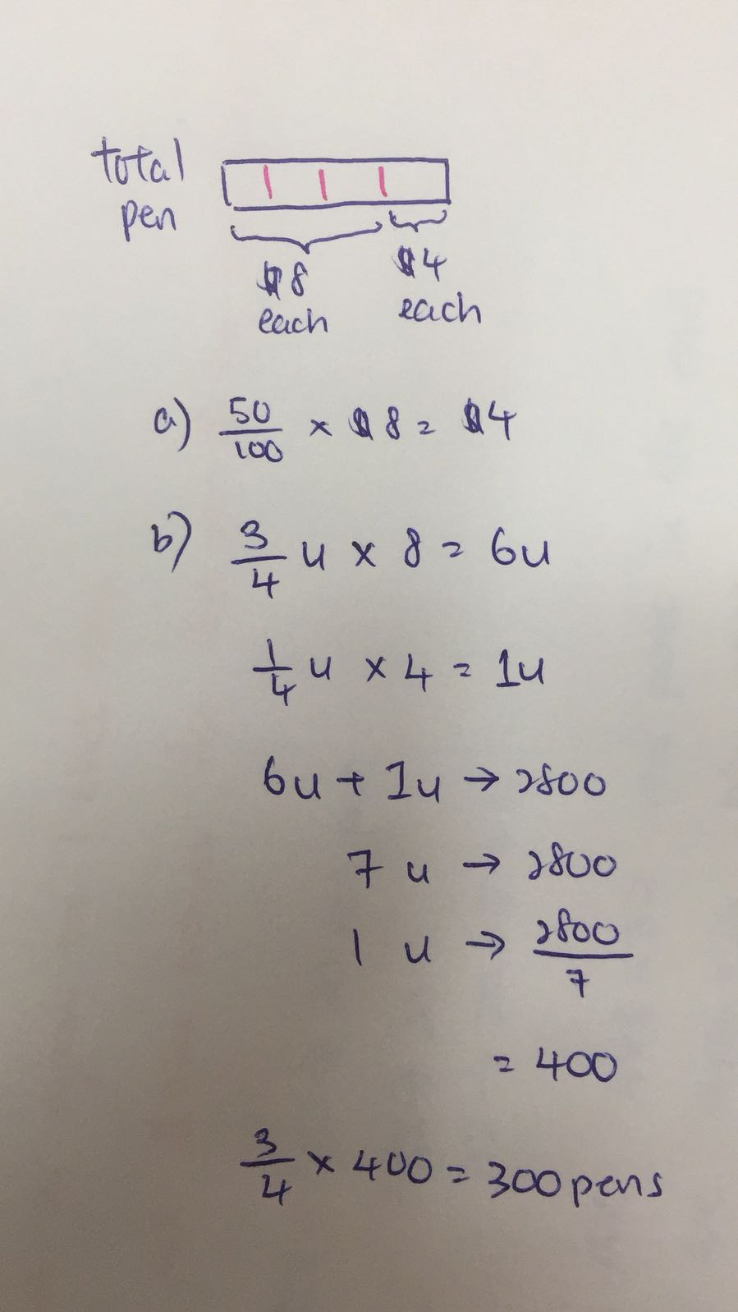# Question

Hi all need help with question, thank you!

Mr Siva sold a number of similar pens. He sold 75% of them at \$8 each and the rest at a discount

of 50%. He collected \$2800 from the sales of these pens.

a) What was the price of 1 pen after the discount?

b) How many pens did Mr Siva sell at \$8 each?0 Replies 1 Like ✔Accepted Answer

(a)
8 x 50% = 4

(b)
75 units x 8 + 25 units x 4 = 700 units = 2800
1 unit = 2800/700 = 4
75 units = 75 x 4 = 300

Ans : (a) \$4; (b) 300 pens.

0 Replies 1 Like